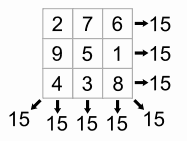Definition of

# Magic SquareA square filled with numbers so that the total of each row, each column and each main diagonal are all the same.

In this example, each row, column and main diagonal add up to 15

The squares can be 3×3 in size, 4×4 and larger.